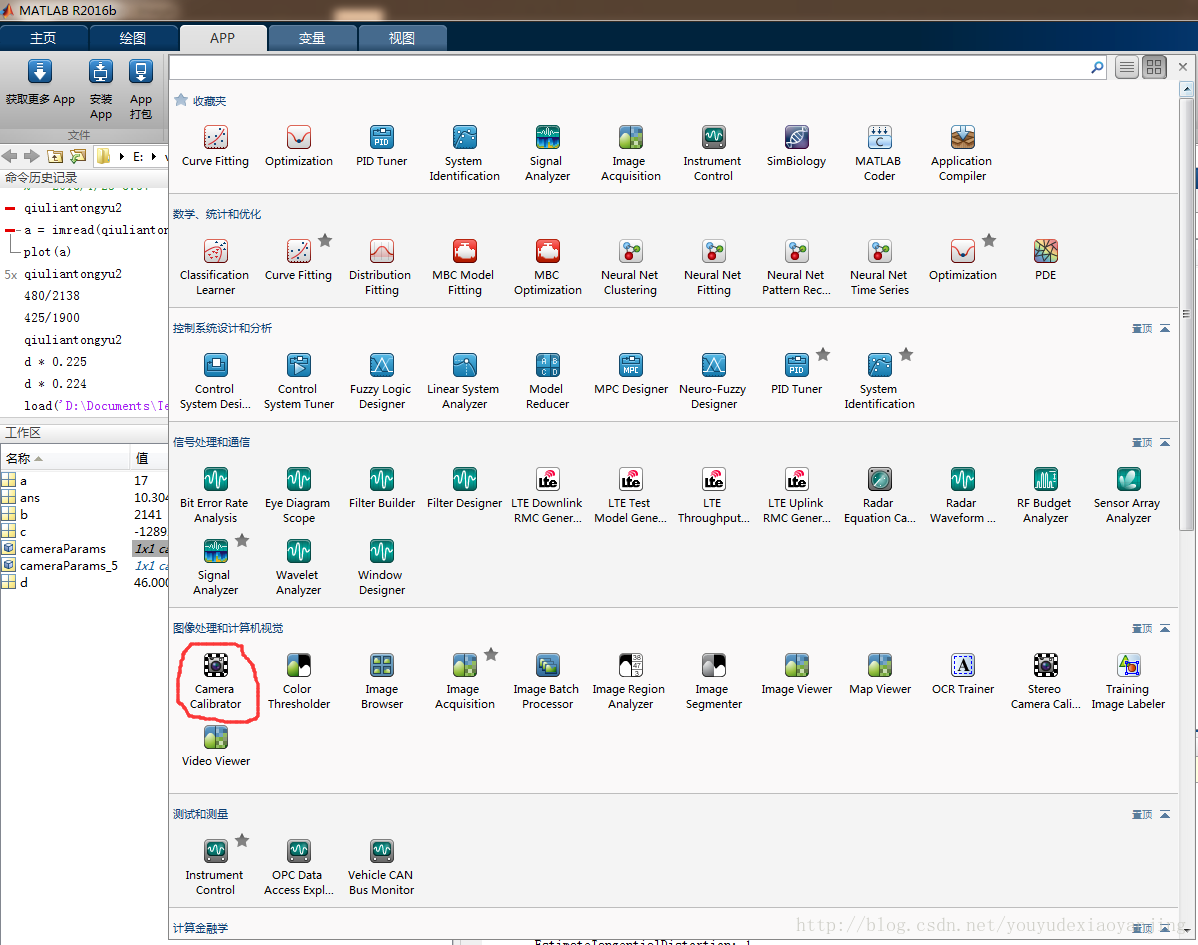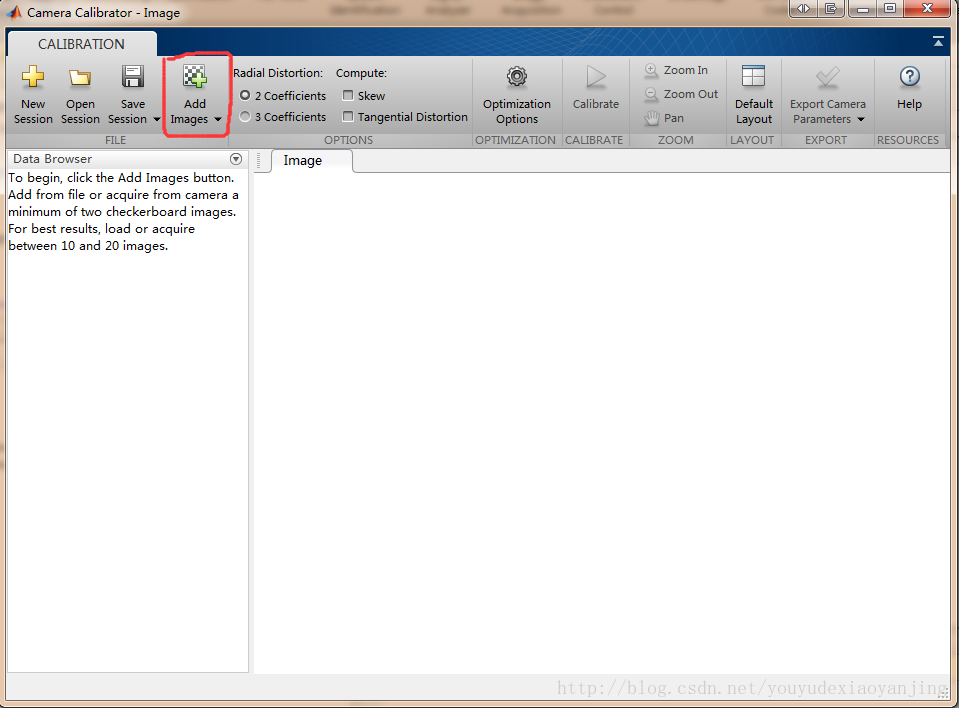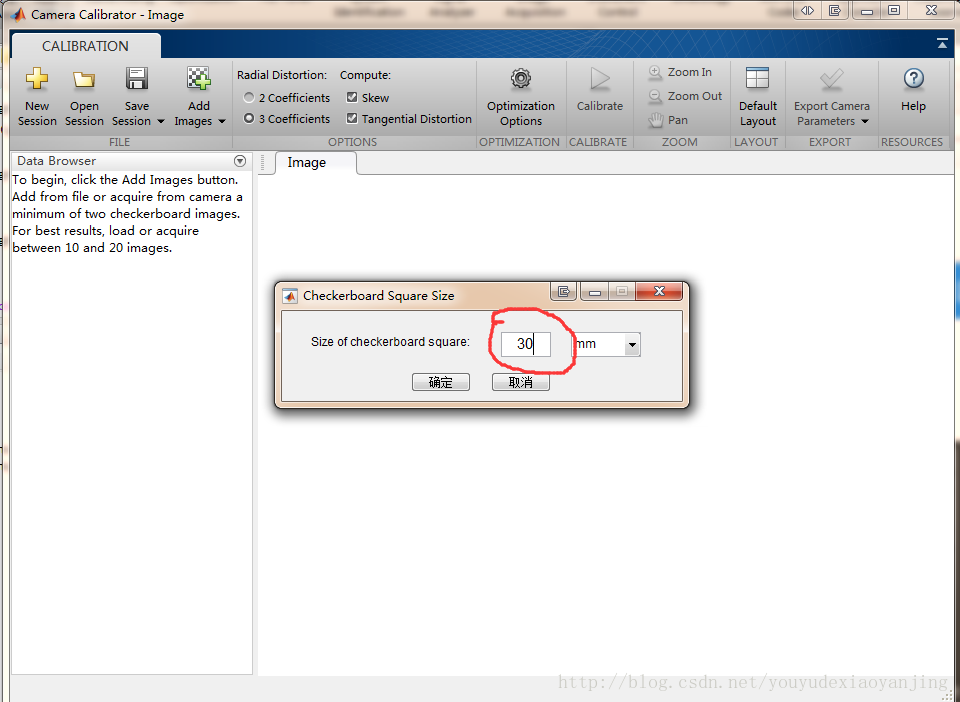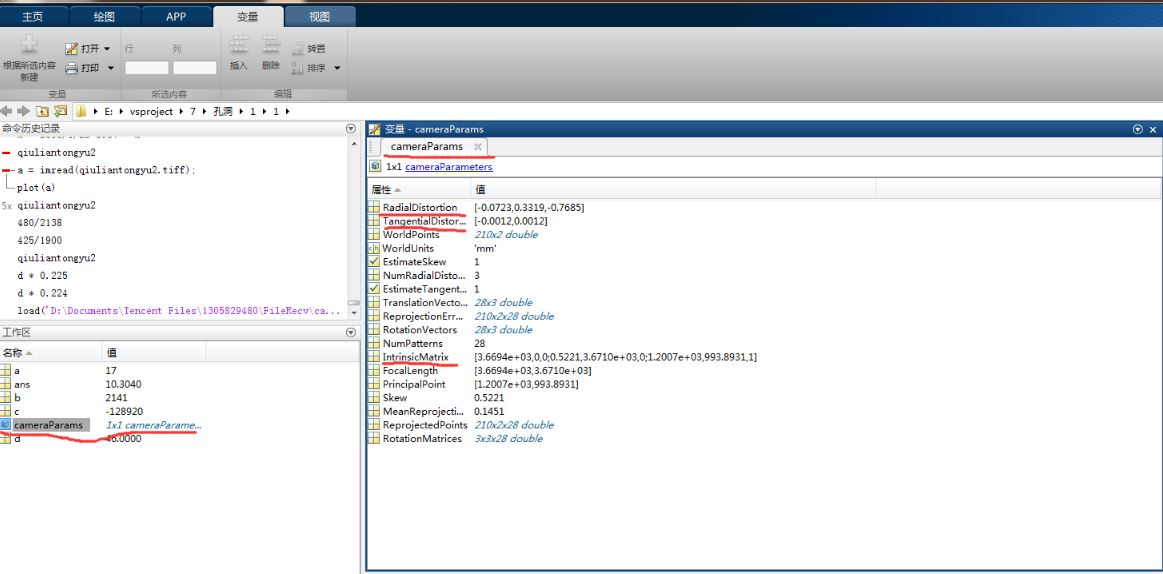# 使用matlab得到校正相机以及如何在opencv里使用

1、打开matlab，如图中红色线条圈出的地方，此处使用matlab2016b：2.打开存放照片的文件夹，全部选中，导入图片3.此处填写标定板方块边长，是指棋盘格每个小格的实际距离4、然后点击上方的Calibrate，处理完后点击Export Camera Parameters（就是绿色对勾）就可以导出数据，可以在matlab的工作区看见，右侧标出的是以后主要用到的数据：{k1 k2 p1 p2 k3 }
matlab中 RadialDistortion 是k1 k2 k3， TangentialDistortion是p1 p2

ans =

955.8925（fx） 0 0
0 955.4439（fy） 0
296.9006（cx） 215.9074（cy） 1.0000

opencv里需要用到内参矩阵和畸变矩阵使用示例：

double fx,fy,cx,cy,k1,k2,k3,p1,p2;
fx=955.8925;
fy=955.4439;
cx=296.9006;
cy=215.9074;
k1=-0.1523;
k2=0.7722;
k3=0;
p1=0;
p2=0;
Mat cameraMatrix = (cv::Mat_<float>(3, 3) <<
fx, 0.0, cx,
0.0, fy, cy,
0.0, 0.0, 1.0);
//    std::cout << cameraMatrix << std::endl;
Mat distCoeffs = (cv::Mat_<float>(5, 1) <<
k1, k2, p1, p2, k3);
//  std::cout << distCoeffs << std::endl;


0 个人打赏©️2019 CSDN 皮肤主题: 技术黑板 设计师: CSDN官方博客